# PROPERTIES OF TRIANGLES, INTERMEDIATE MATHEMATICS FIRST YEAR PROBLEMS WITH SOLUTIONS

Intermediate mathematics first year 1A and 1B some chapters. The solutions are very easy to understand.

Inter first year 1A : Functions, mathematical induction, functions, addition of vectors, trigonometric ratios upto transformations, trigonometric equations, hyperbolic functions, inverse trigonometric functions and properties of triangles.

Inter first year 1B : Solutions for locus, transformation of axes and three dimensional coordinates, the straight line, pair of straight lines, the planes and applications of derivatives.

You can see the solutions for junior inter maths 1A

3. Matrices

You can see the solutions for text book Maths 1A

Functions

Exercise 1(a)

Exercise 1(b)

Exercise 1(c)

Mathematical Induction

Exercise 2(a)

Matrices

Exercise 3(a)

Exercise 3(b)

Exercise 3(c)

Exercise 3(d)

Exercise 3(e)

Exercise 3(f)

Exercise 3(g)

Exercise 3(h)

Exercise 3(i)

Exercise 4(a)

Exercise 4(b)

Product of Vectors

Exercise 5(a)

Exercise 5(b)

Exercise 5(c)

Trigonometric Ratios up to Transformations

Exercise 6(a)

Exercise 6(b)

Exercise 6(c)

Exercise 6(d)

Exercise 6(e)

Exercise 6(f)

Trigonometric Equations

Exercise 7(a)

Inverse Trigonometric Equations

Exercise 8(a)

Hyperbolic Functions

Exercise 9(a)

Properties of Triangles

Exercise 10(a)

Exercise 10(b)

Inter Maths Trigonometry text book solutions

Maths 1B solutions for

Locus

Exercise 1(a)

Transformation of Axes

Exercise 2(a)

Three Dimensional Coordinates

Exercise 5(a)

Exercise 5(b)

The Plane

Exercise 7(a)

Limits and Continuity

Exercise 8(a)

Exercise 8(b)

Exercise 8(d)

Exercise 8(e)

M

Junior inter 1B solutions for examination purpose

1Locus

Straight lines sa

Straight lines la

12. Rate measure

Ncert maths class 6 chapter 14 solutions

Nios maths 311 book 1 Sets 1.1 solutions

## SOLUTIONS OF PROPERTIES OF TRIANGLES, JUNIOR INTER TRIGONOMETRY

### Properties of Triangles solutions, Intermediate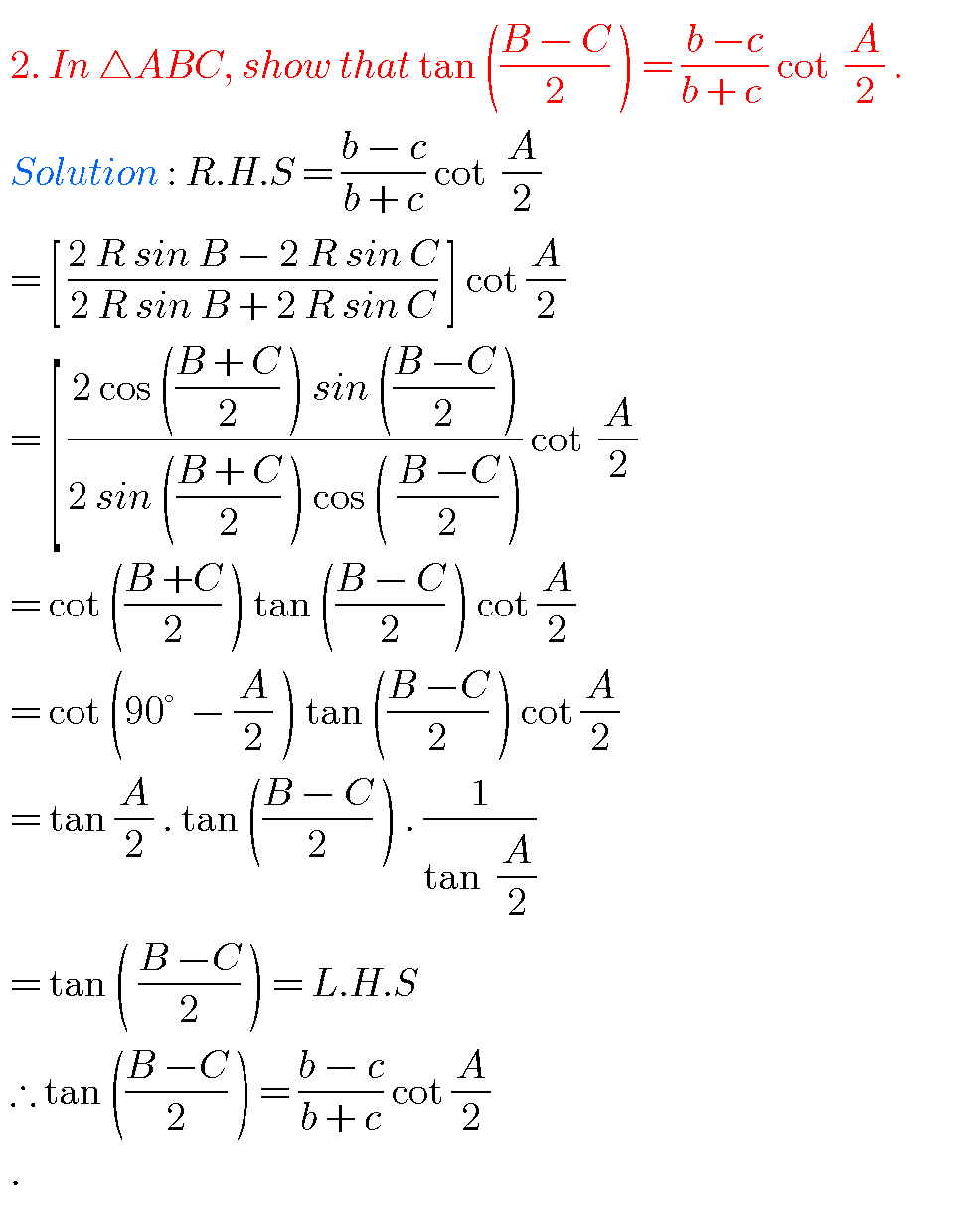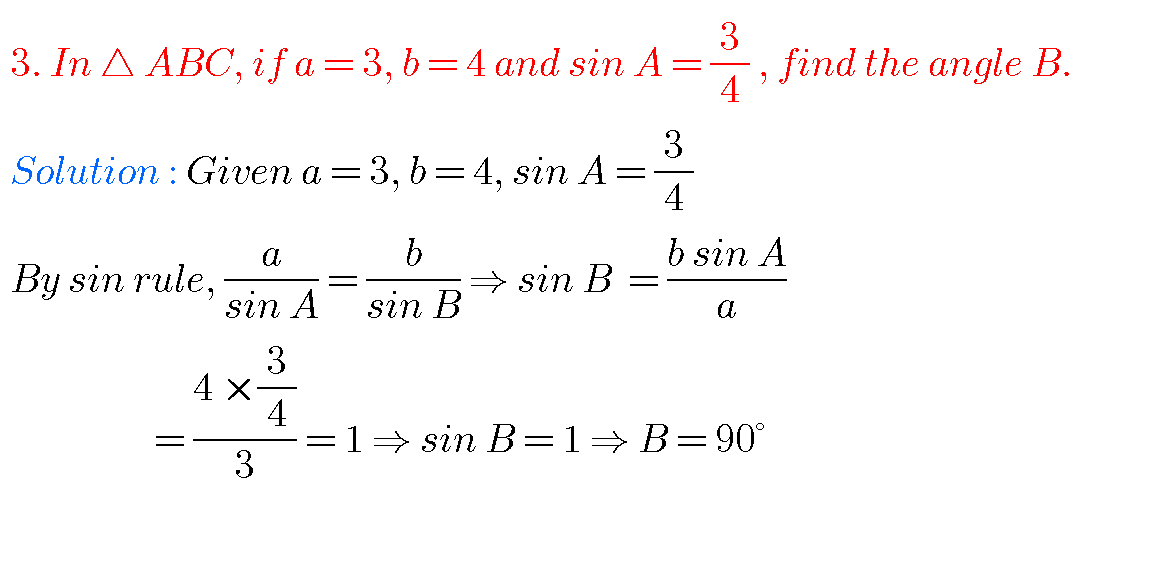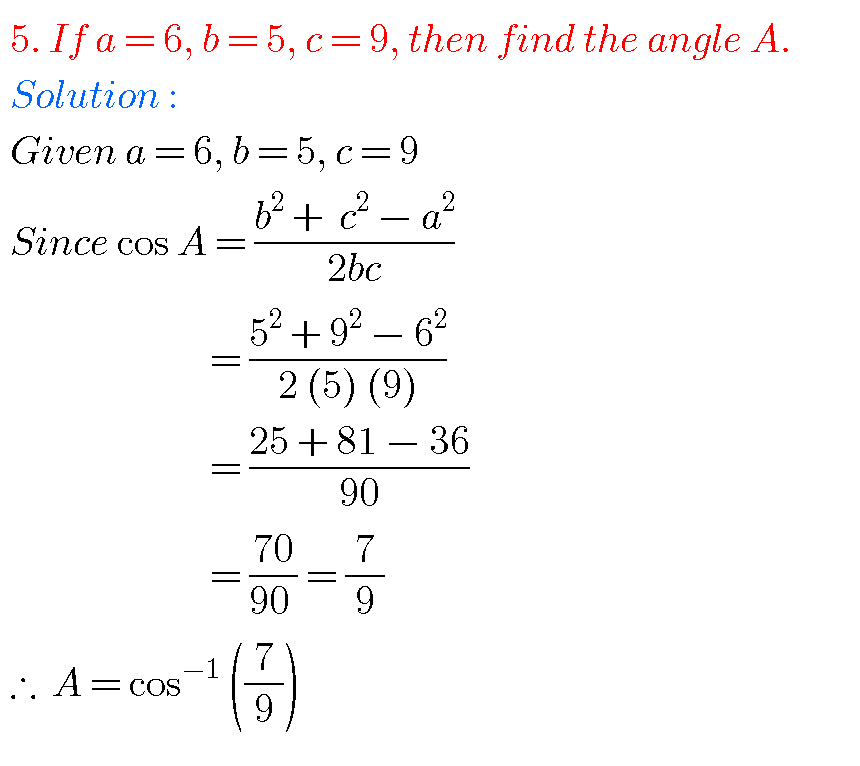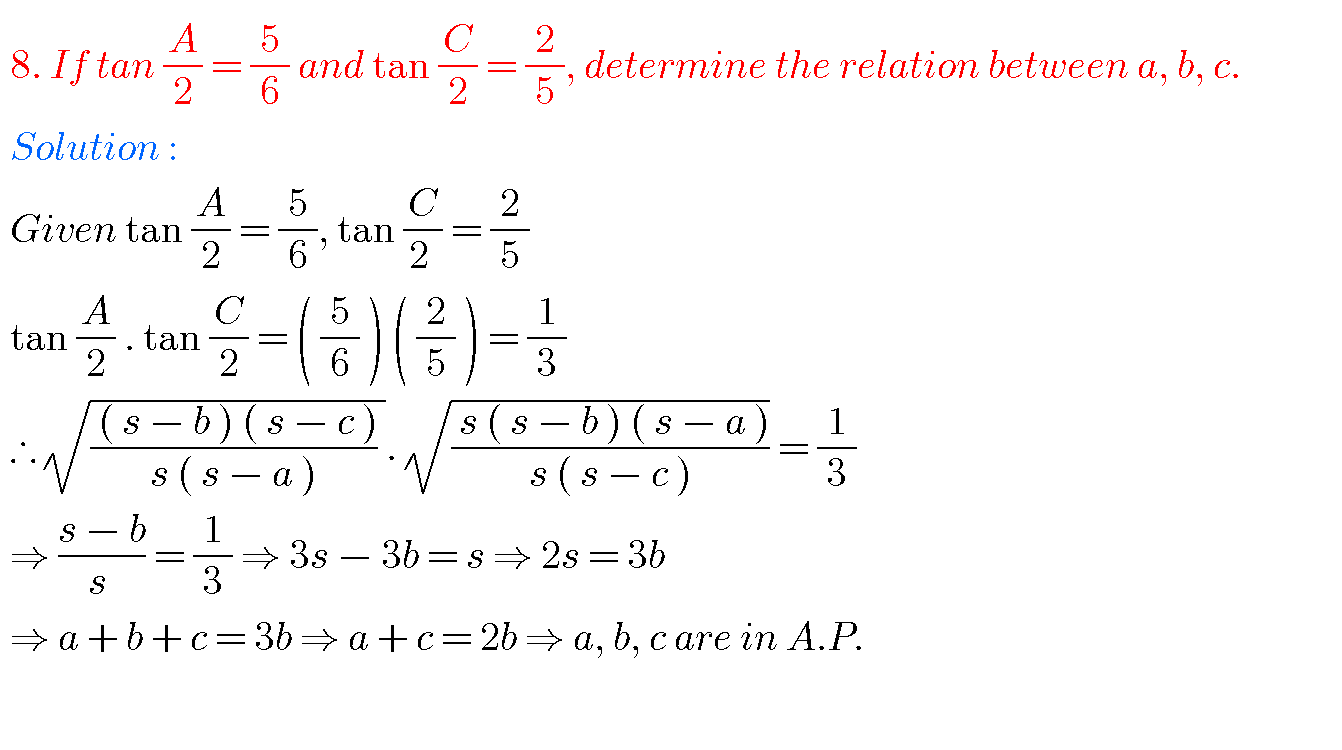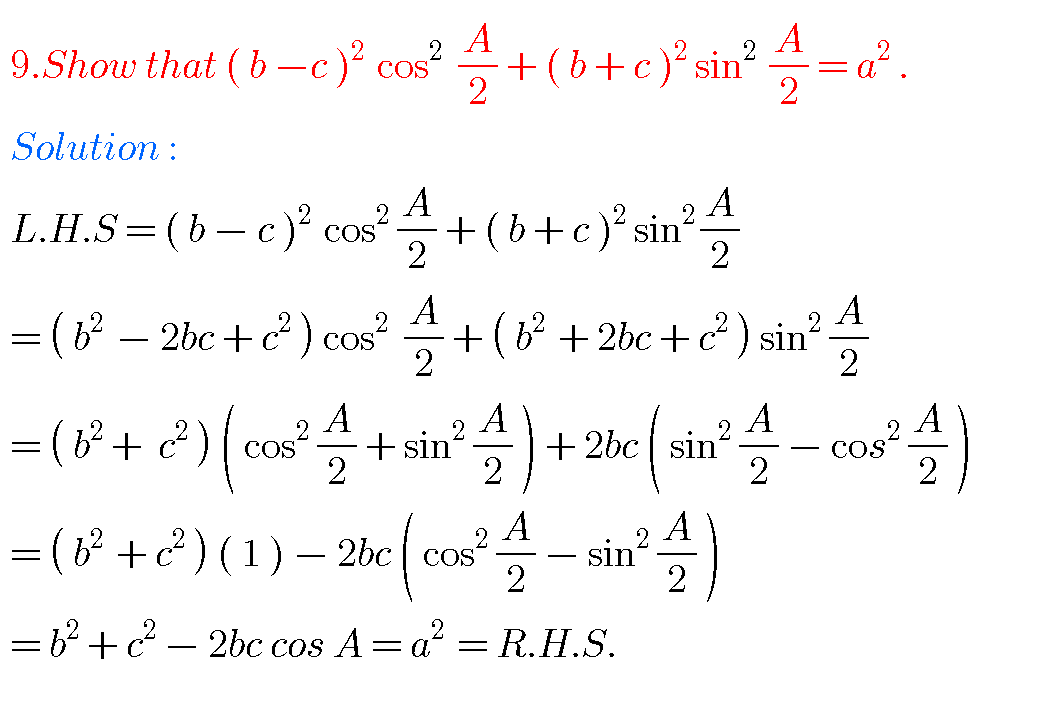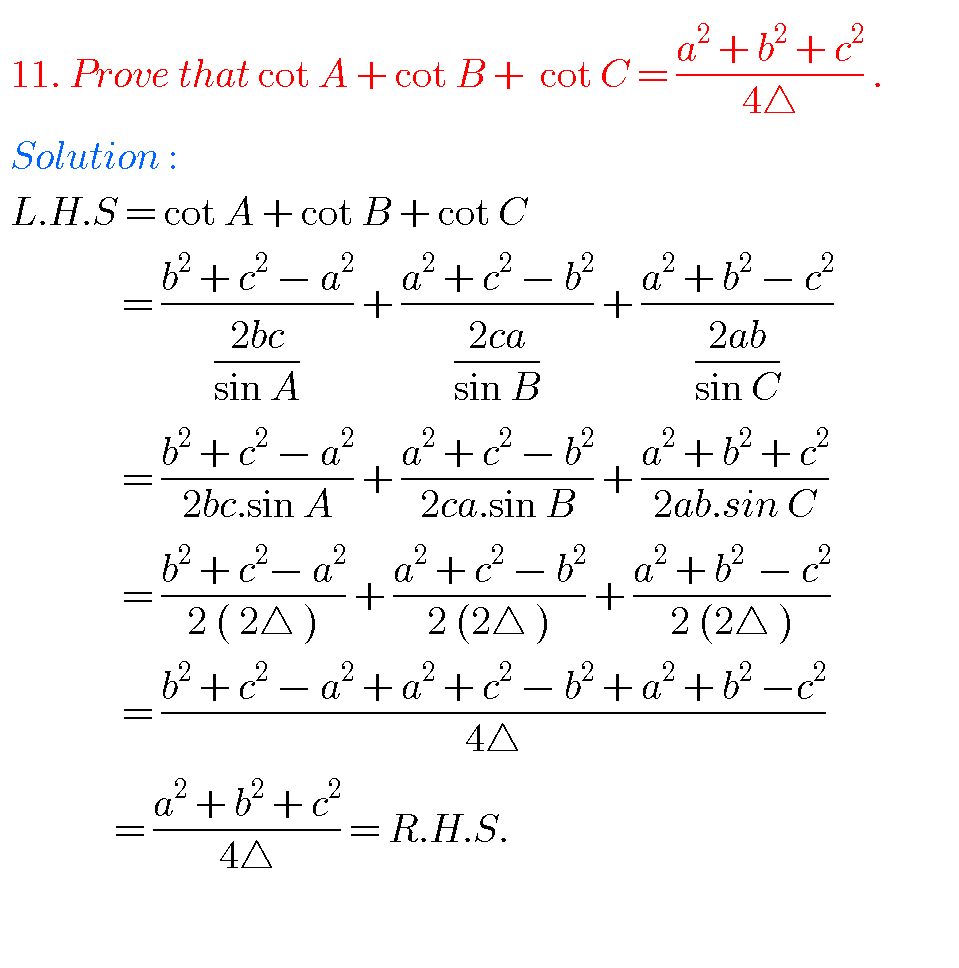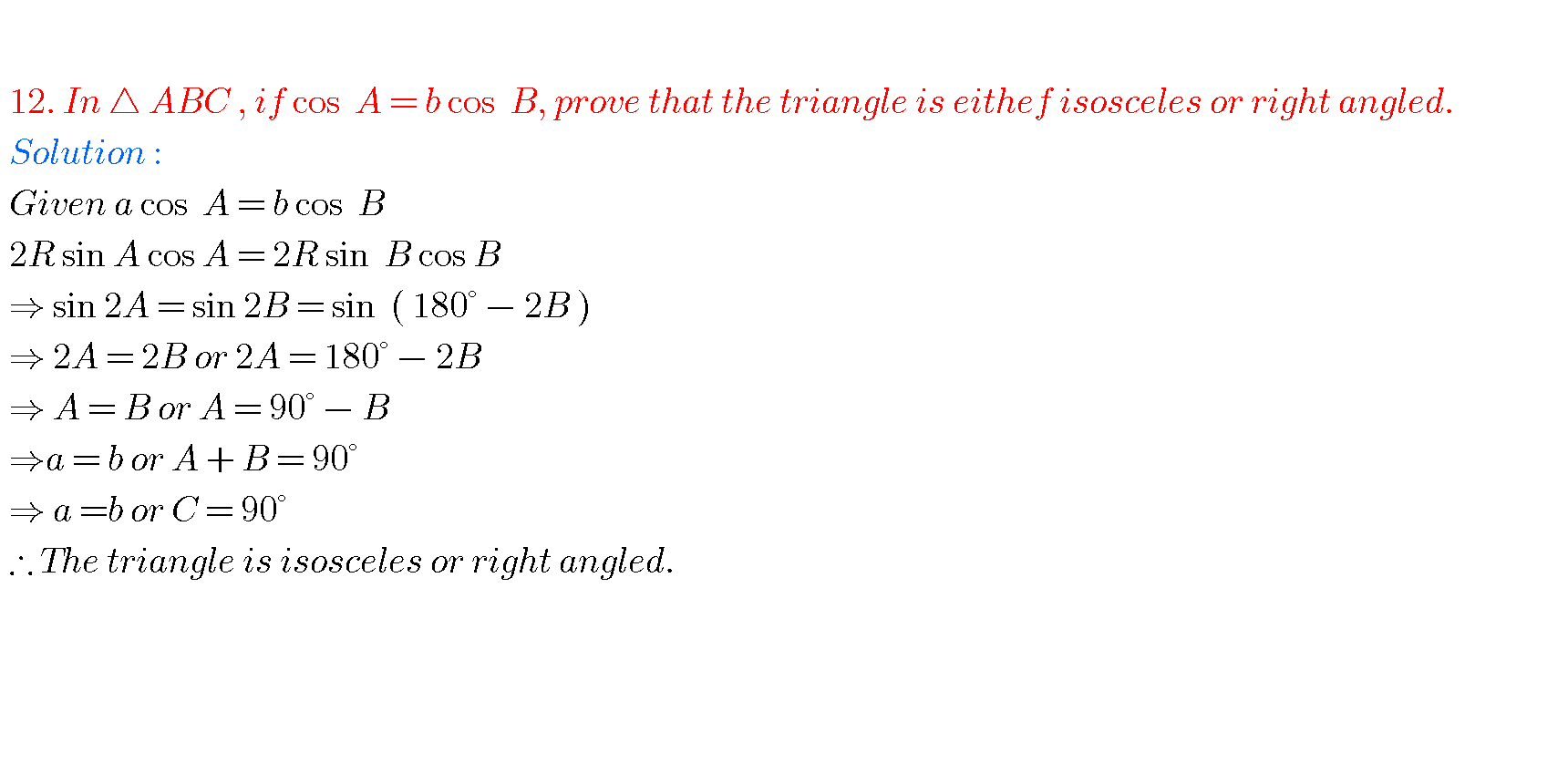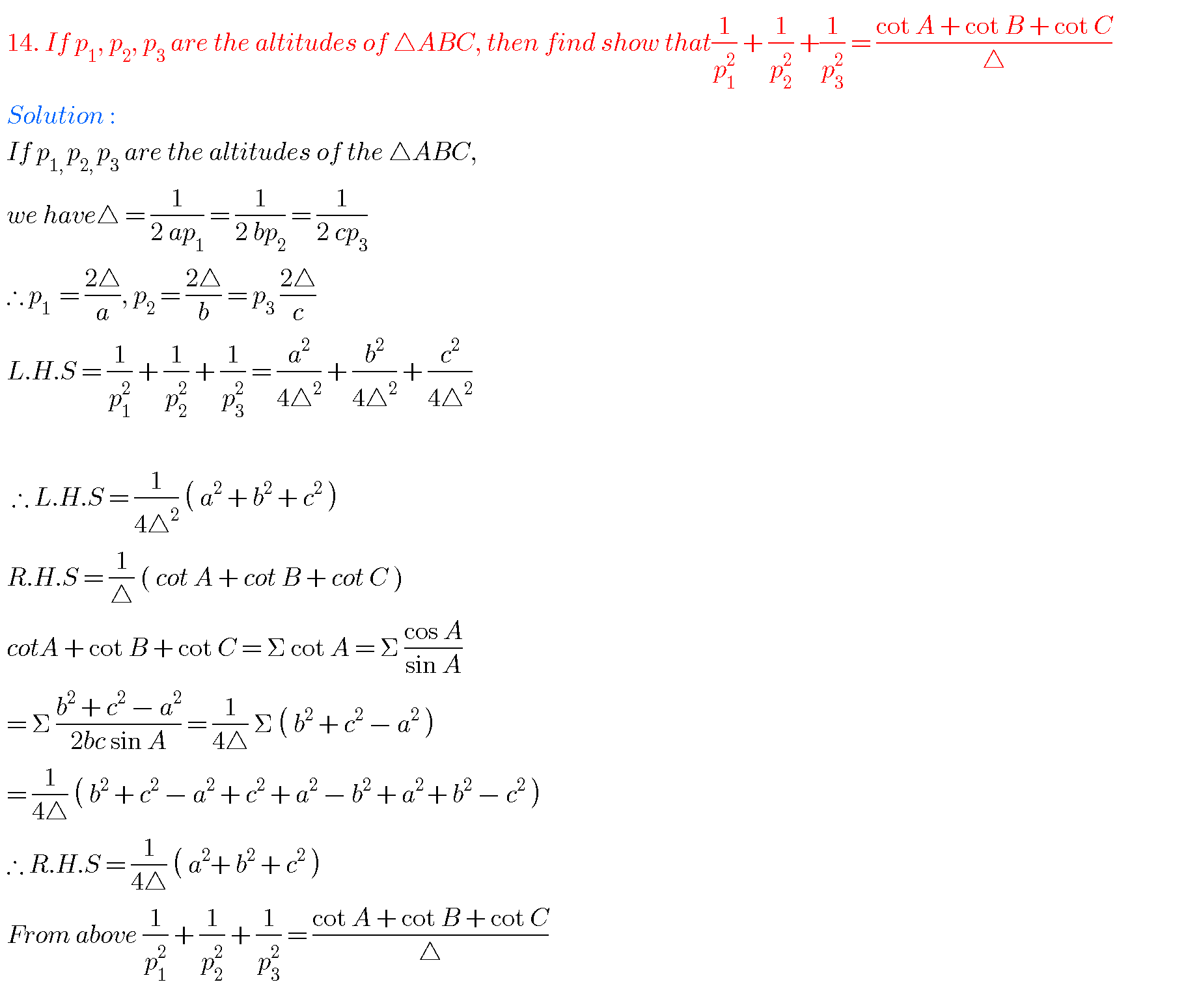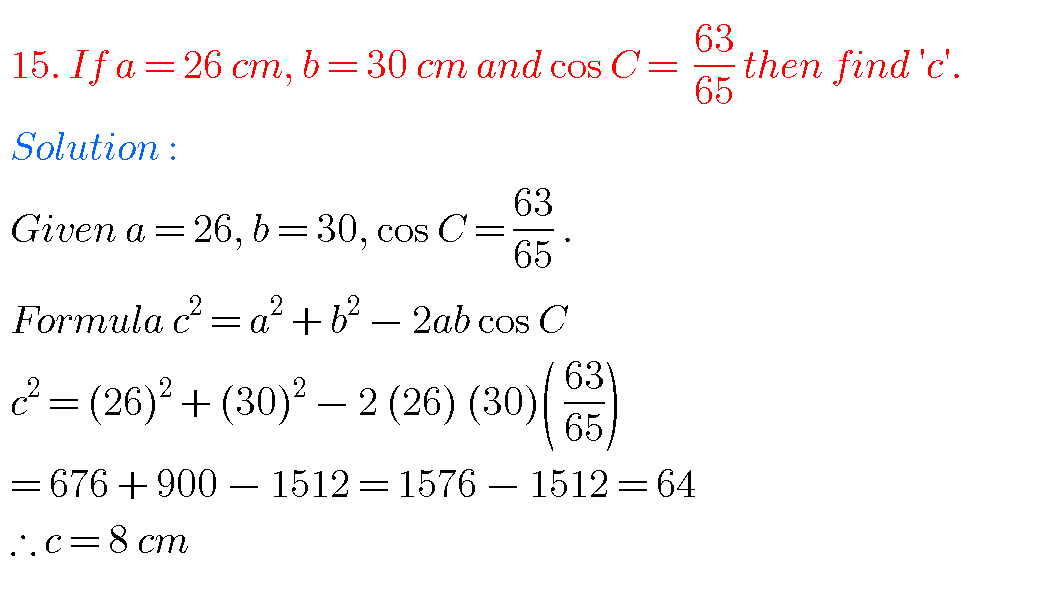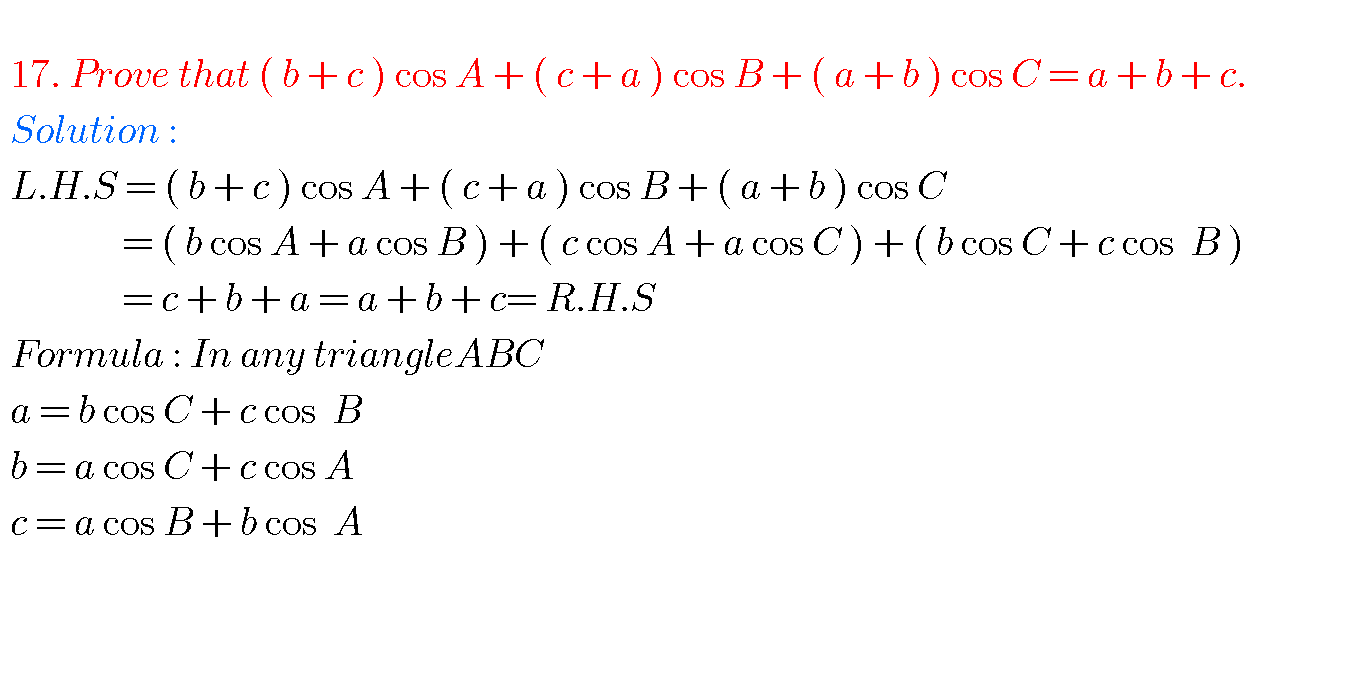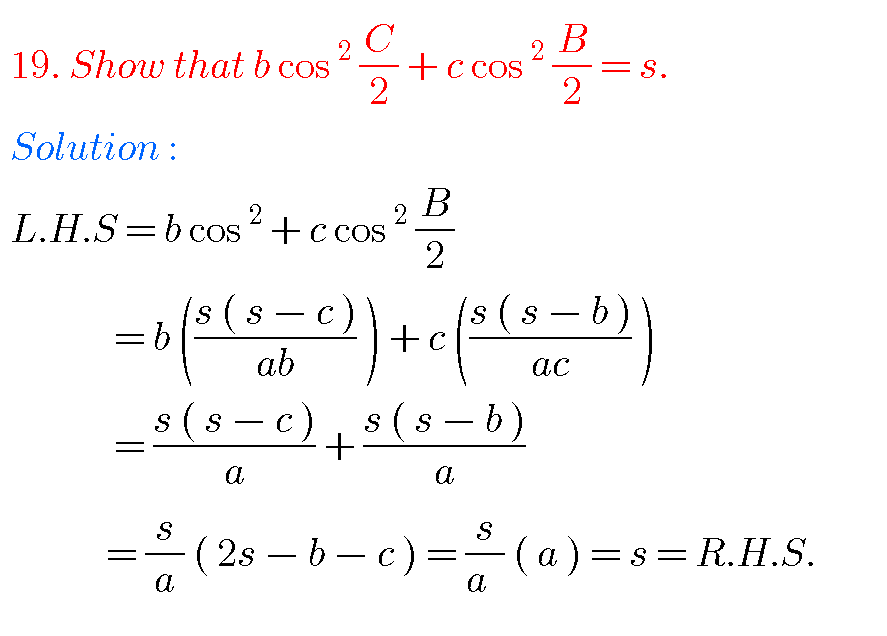Problem 21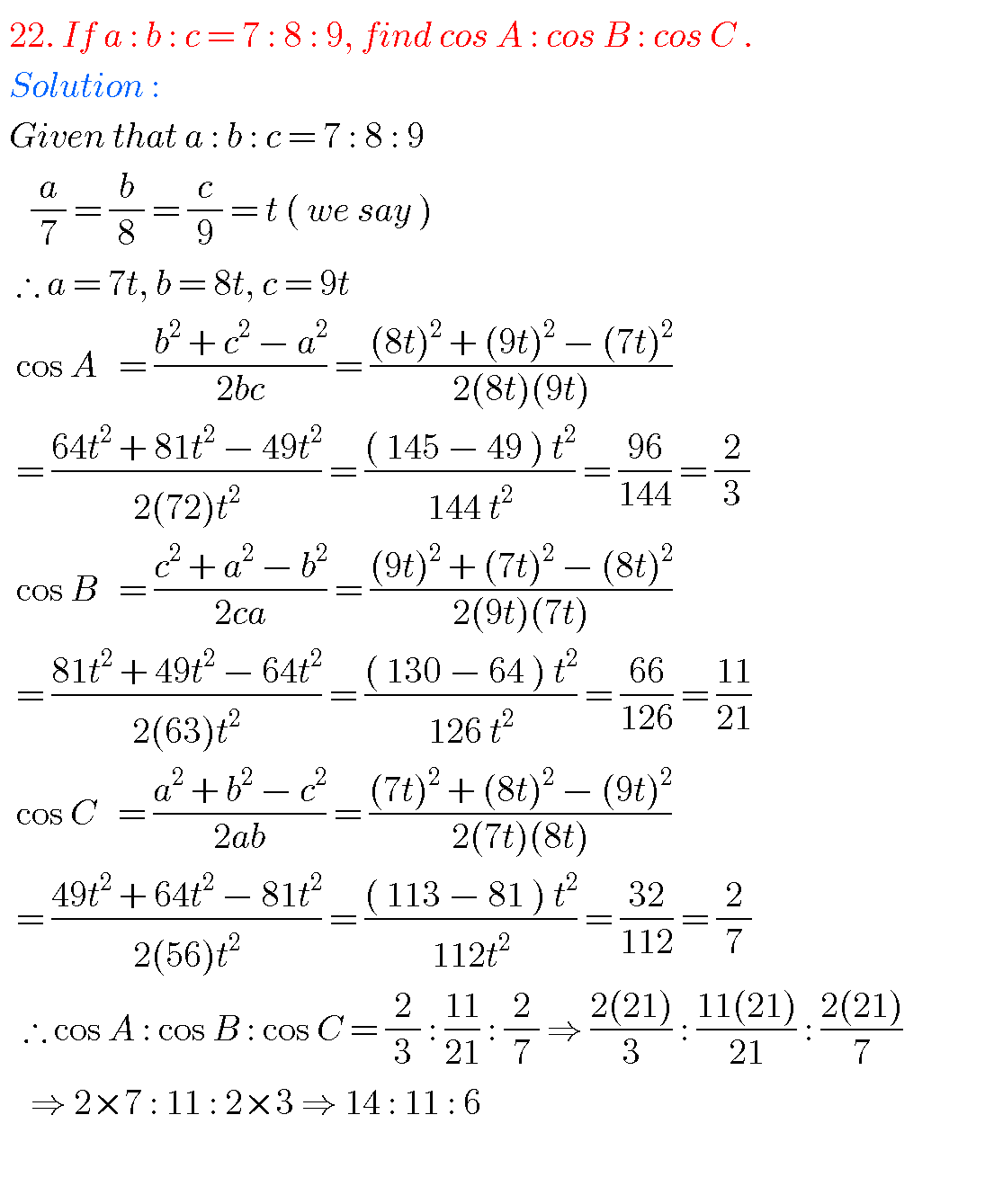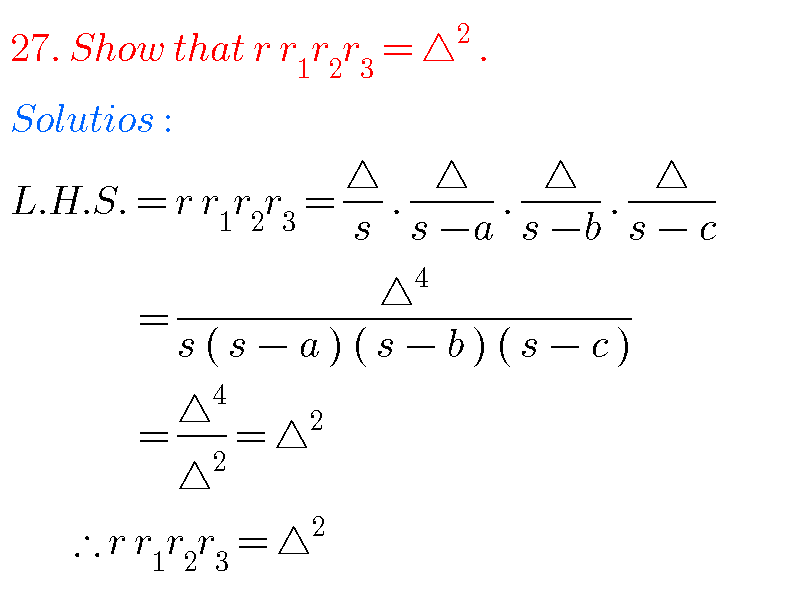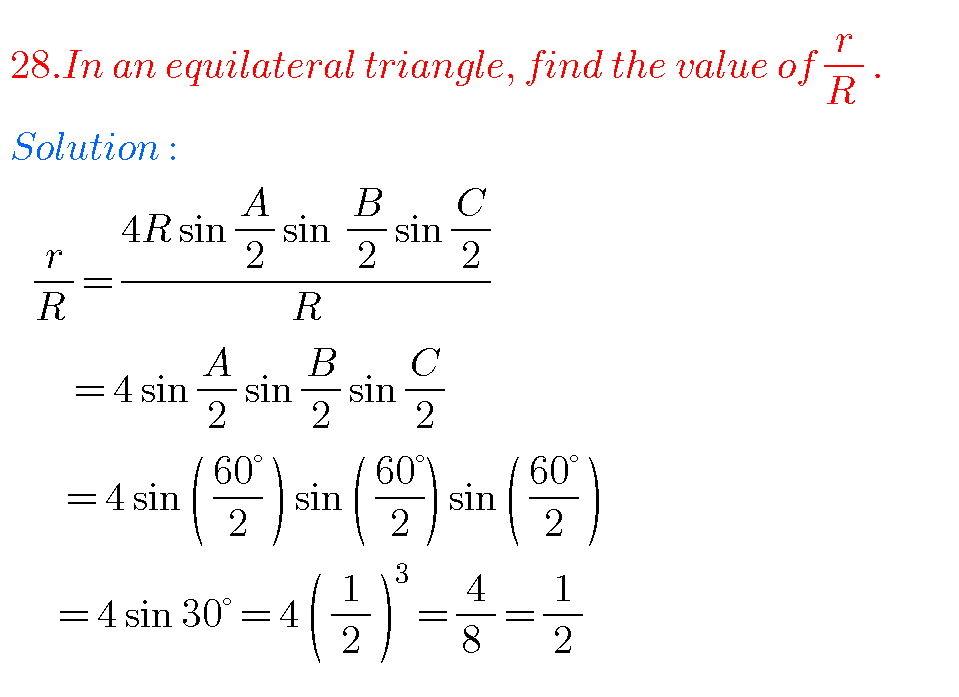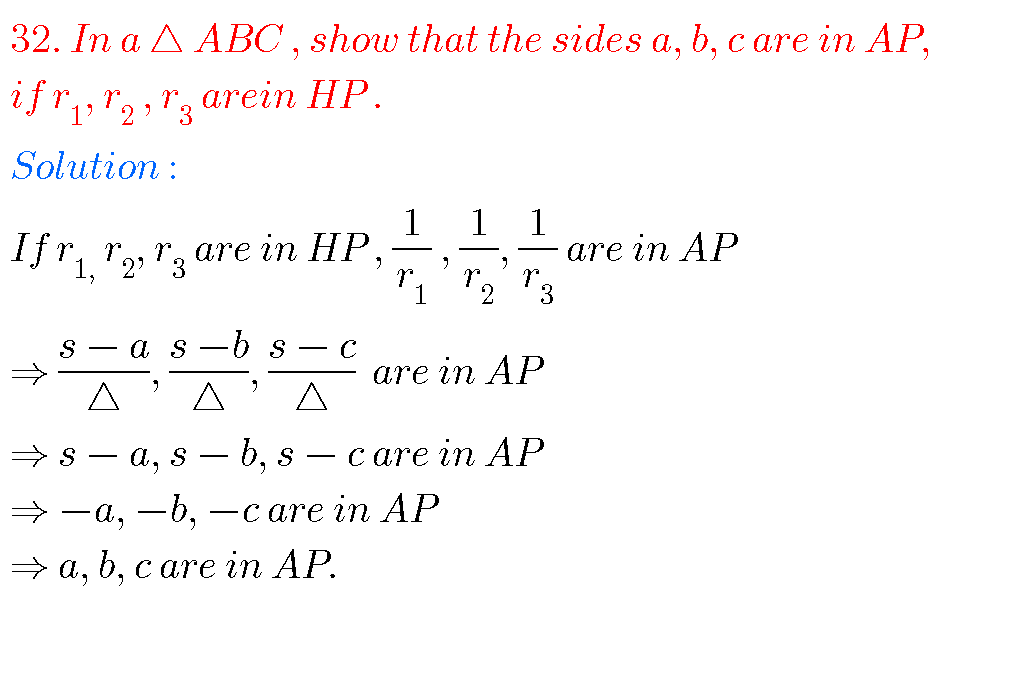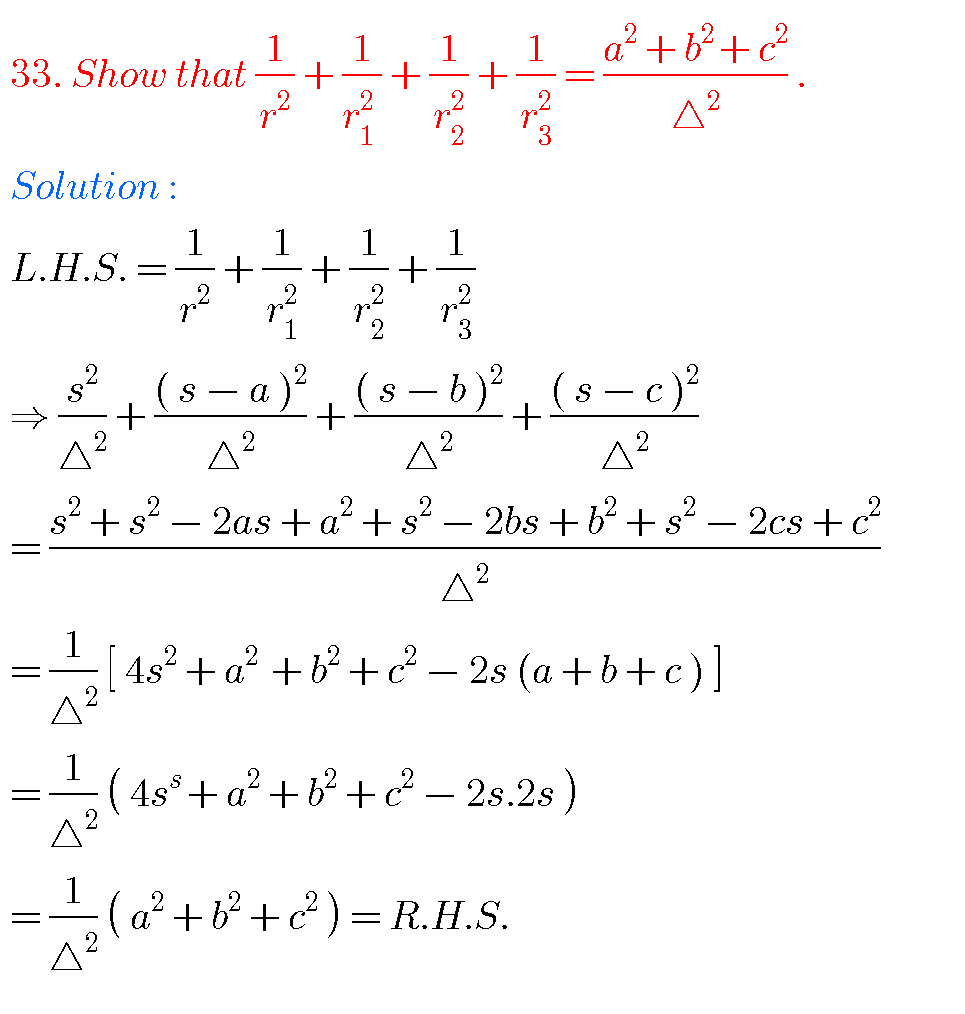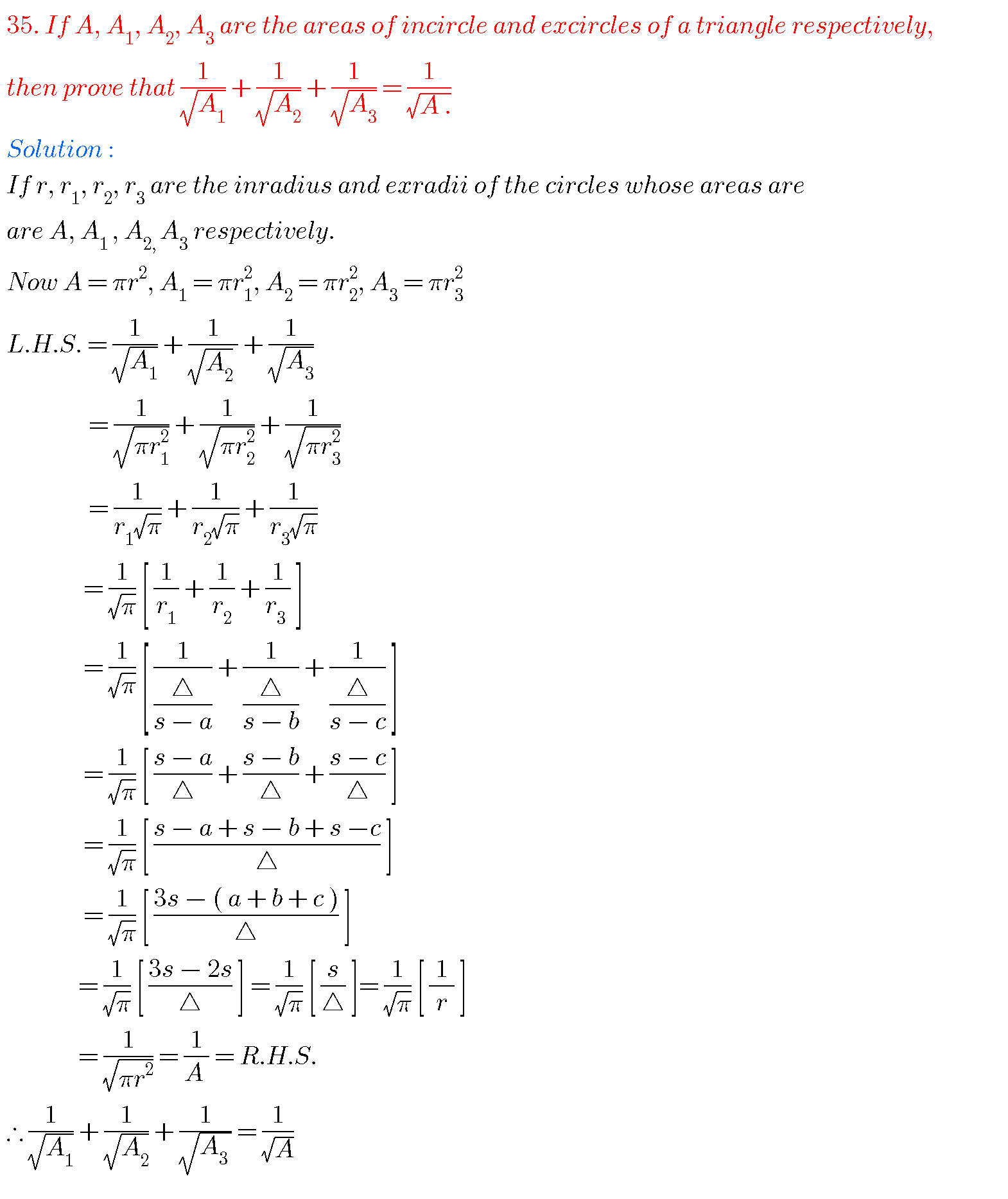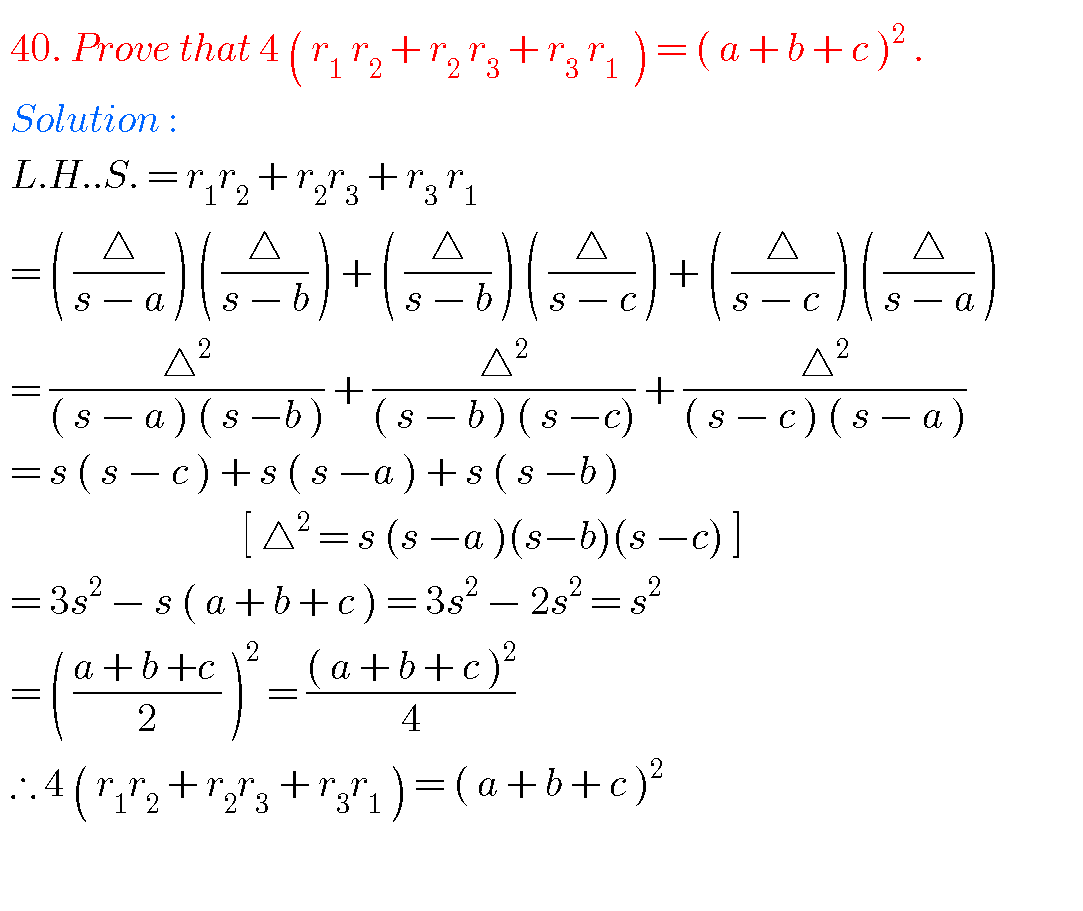# SOLUTIONS OF PROPERTIES OF TRIANGLES, JUNIOR INTER TRIGONOMETRY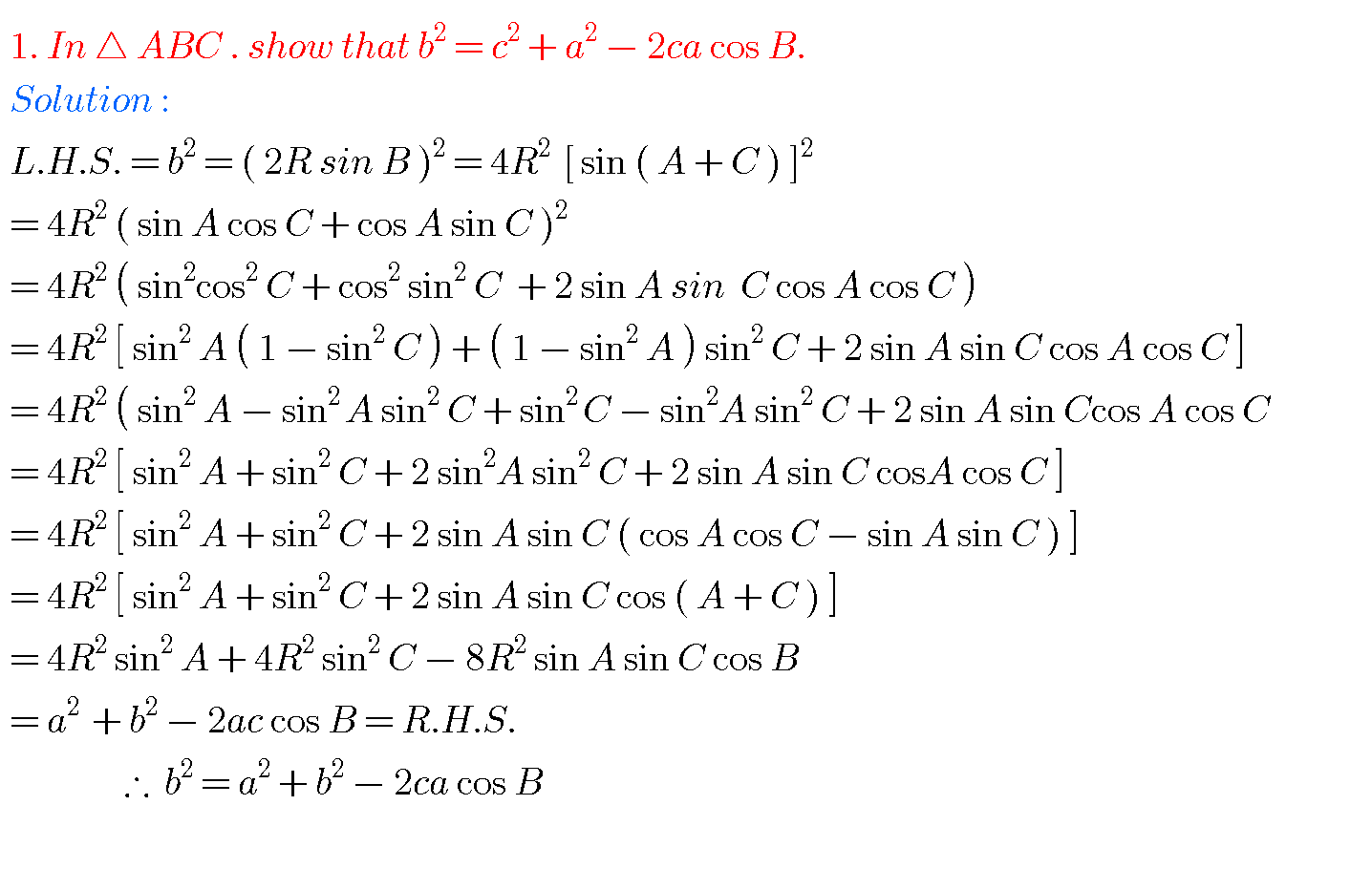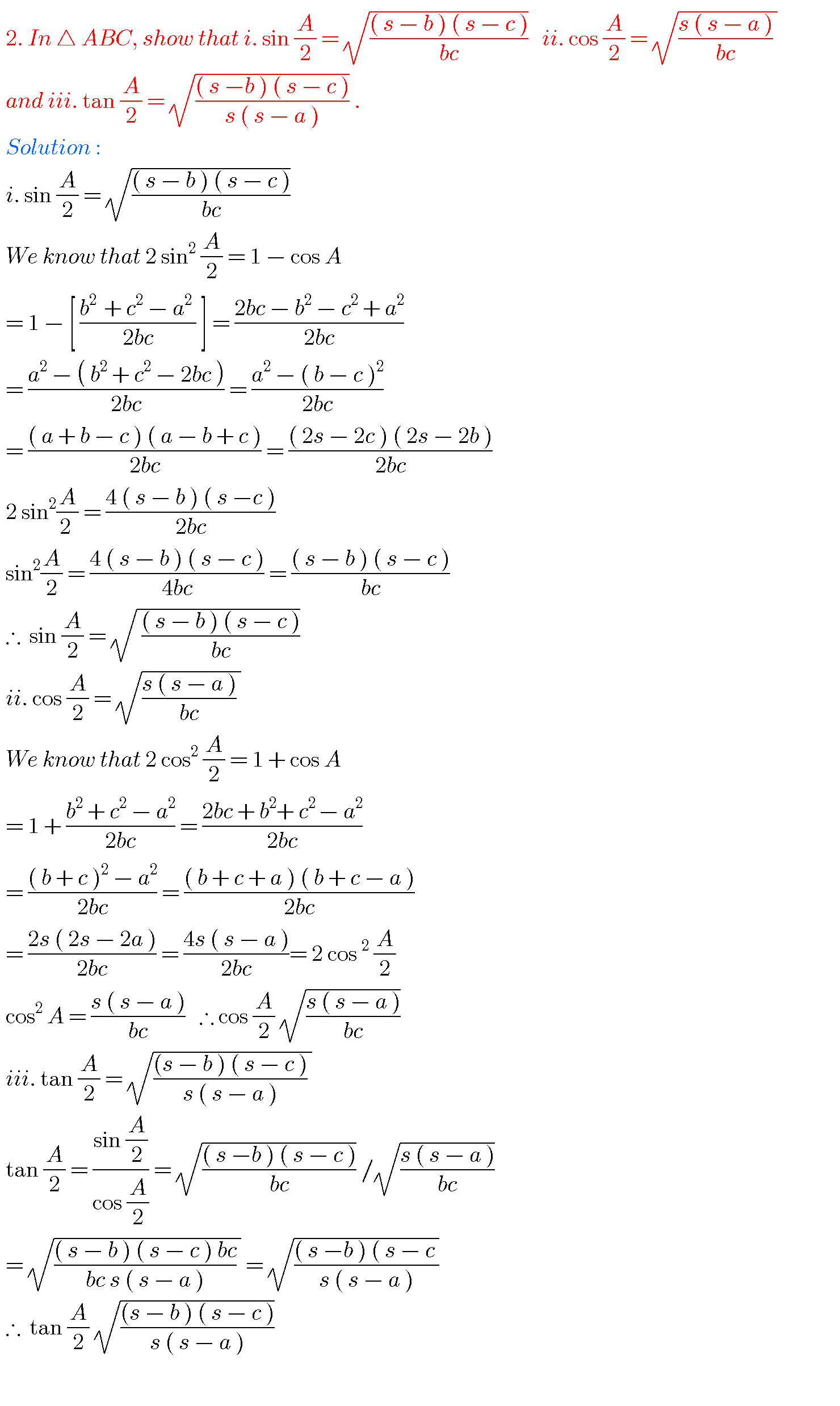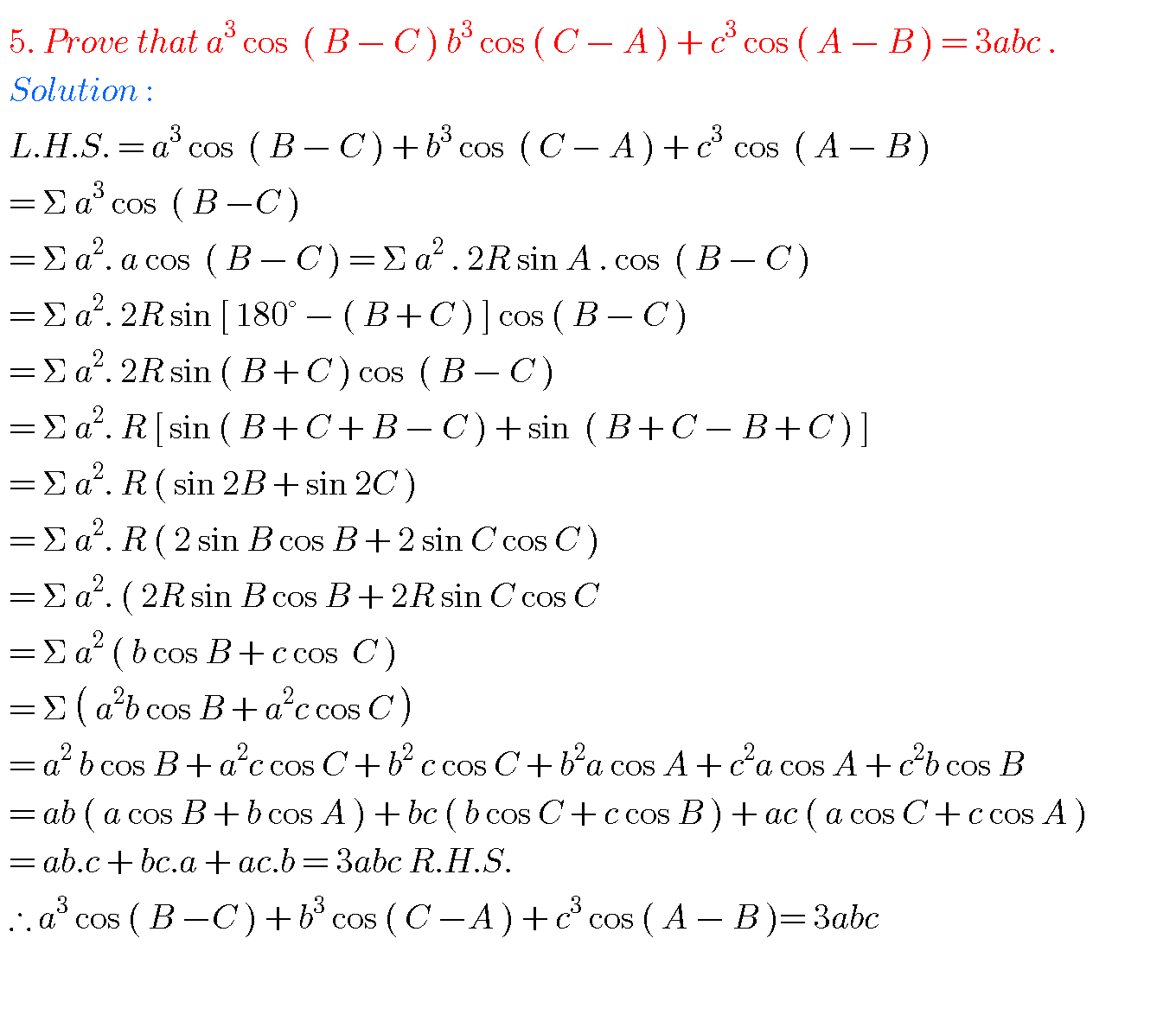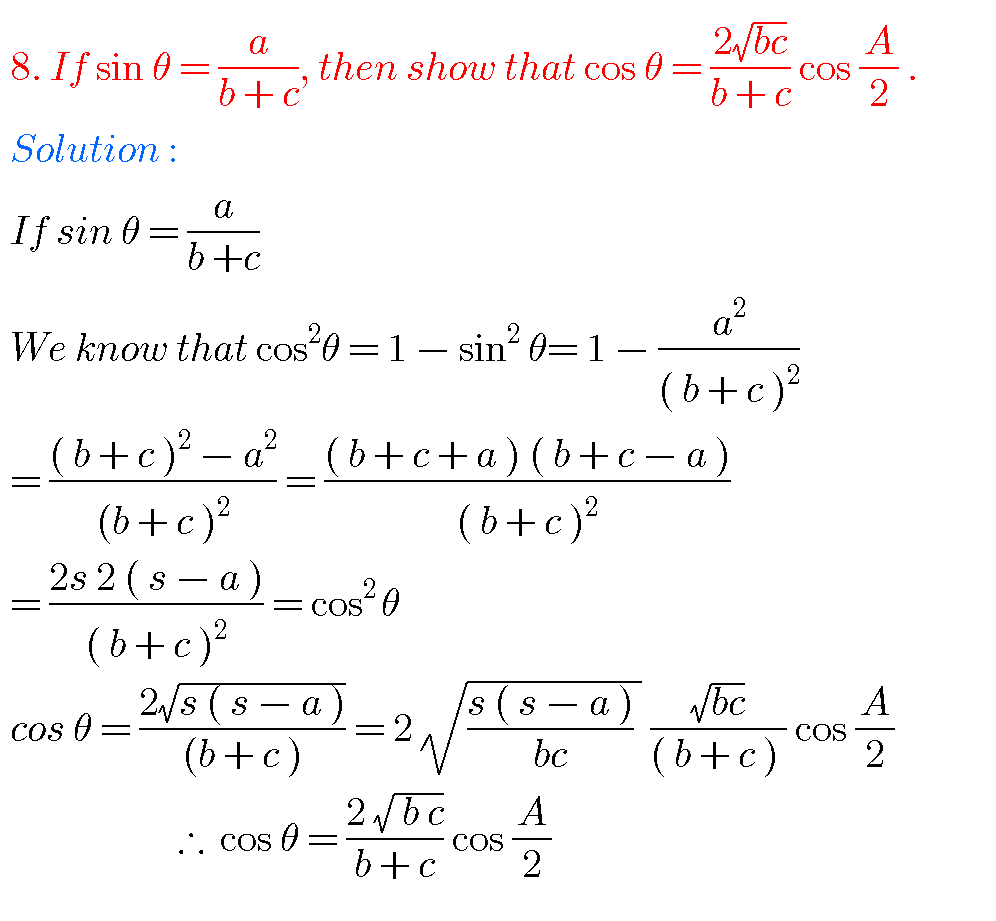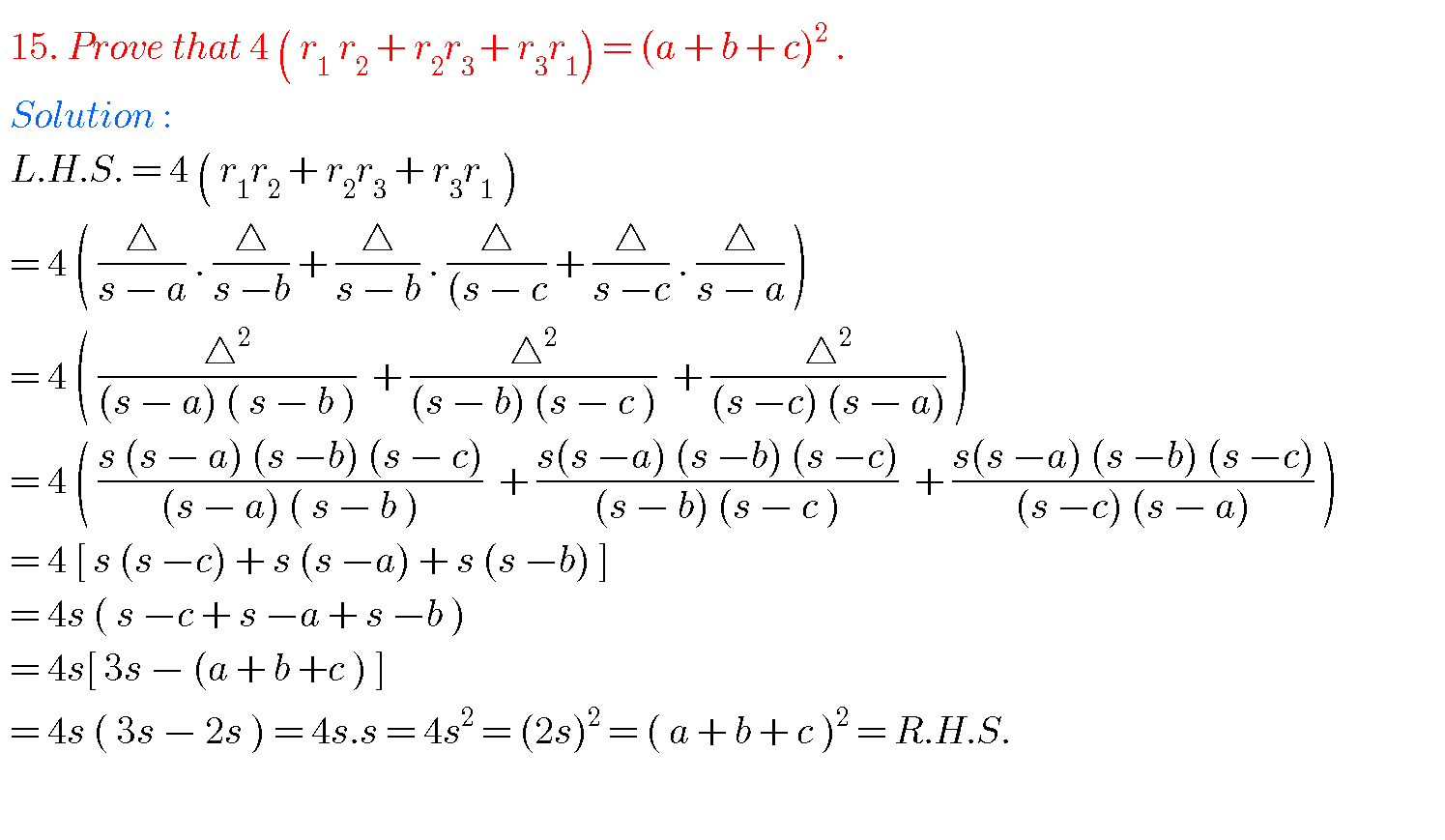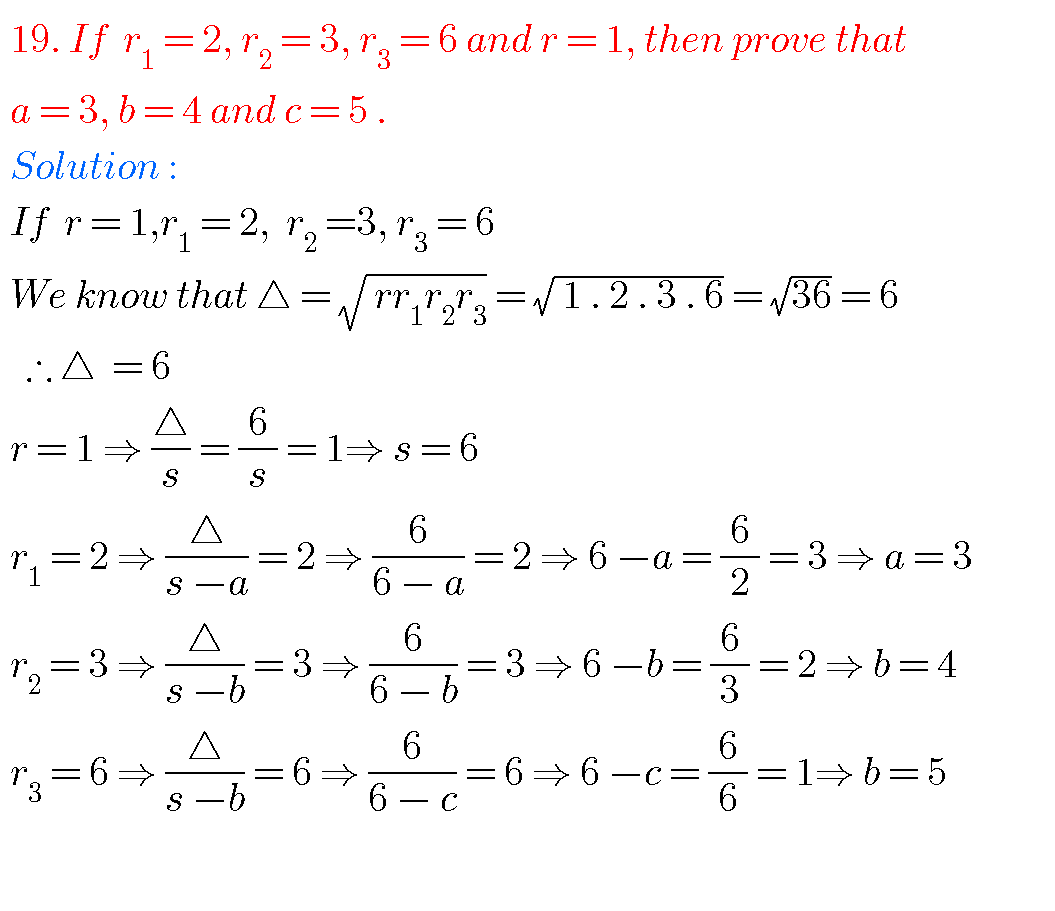### PROPERTIES OF TRIANGLES

Note : Observe the solutions of properties of triangles and try them in your own methods.

Ncert solutions for maths class 6 some chapters

Ncert solutions for maths class 7 some chapters

Ncert solutions for maths class 8 some chapters

Ncert solutions for class 8 chapter 12

Maths solutions class 10

You can see text book solutions for Inter Maths IIB

1. Circle

3. Parabola

4. Ellipse

You can also see solutions for Inter Maths text book IIA

For examination purpose you can see

Complex numbers

De Moivre’ s Theorem

### One Comment

1.Khader October 30, 2019 at 11:07 am - Reply

Hello I am not able to see Maths A questions and answers